# Writing Fractions as Percents

## Learn About Writing Fractions as Percents With The Following Examples And Interactive ExercisesProblem: Last marking period, Ms. Jones gave an A grade to 15 out of every 100 students and Mr. McNeil gave an A grade to 3 out of every 20 students. What percent of each teacher's students received an A?

 Solution Teacher Ratio Fraction Percent Ms. Jones 15 to 100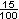15% Mr. McNeil 3 to 2015%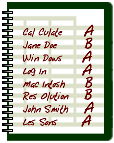Solution: Both teachers gave 15% of their students an A last marking period.

In the problem above, the fraction for Ms. Jones was easily converted to a percent. This is because It is easy to convert a fraction to a percent when the denominator is 100. If a fraction does not have a denominator of 100, you can convert it to an equivalent fraction with a denominator of 100, and then write the equivalent fraction as a percent. This is what was done in the problem above for Mr. McNeil. Let's look at some problems in which we use equivalent fractions to help us convert a fraction to a percent.

Example 1: Write each fraction as a percent: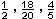Solution Fraction Equivalent Fraction Percent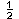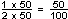50%90%80%

Example 2: One team won 19 out of every 20 games played, and a second team won 7 out of every 8 games played. Which team has a higher percentage of wins?

 Solution Team Fraction Equivalent Fraction Percent 1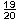95% 2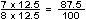87.5%

Solution: The first team has a higher percentage of wins.

In Examples 1 and 2, we used equivalent fractions to help us convert each fraction to a percent. Another way to do this is to convert each fraction to a decimal, and then convert each decimal to a percent. To convert a fraction to a decimal, divide its numerator by its denominatorLook at Example 3 below to see how this is done.

Example 3: Write each fraction as a percent: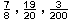Solution Fraction Decimal Percent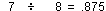87.5%95%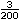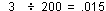1.5%

Now that you are familiar with writing fractions as percents, do you see a pattern in the problem below?

 Problem: If 165% equals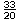, and 16.5% equals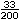, then what fraction is equal to 1.65%?
 Solution Percent Fraction 165%16.5%1.65%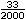Summary: To write a fraction as a percent, we can convert it to an equivalent fraction with a denominator of 100. Another way to write a fraction as a percent is to divide its numerator by its denominator, then convert the resulting decimal to a percent.

### Exercises

Directions: Read each question below. Select your answer by clicking on its button. Feedback to your answer is provided in the RESULTS BOX. If you make a mistake, choose a different button.

 1.  Which of the following is equal to 36%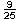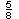None of the above.       RESULTS BOX:
 2.  Which of the following is equal to 62.5%?None of the above.       RESULTS BOX:
 3.  Which of the following is equal to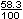? .583%       5.83%       58.3%       None of the above.       RESULTS BOX:
 4.  Which of the following is equal to? 11%       5.5%       200%       None of the above.       RESULTS BOX:
 5.  What fraction is equal to .42%?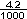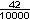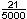All of the above.       RESULTS BOX: